These functions extend autoplot.sp() and autoplot.elev() and return not a single plot but a list of plots. They are particularly useful if you want to print a .pdf file with one plot per page. They automatically plot the variables sp and elev of a ForestGEO-like dataset of class 'sp' or 'sp_elev'.

• Create a 'sp' object with:

    object <- sp(DATA-WITH-VARIABLE-sp)

• Create a 'sp_elev' object with:

    object <- sp_elev(DATA-WITH-VARIABLE-sp, DATA-WITH-VARIABLE-elev)


See sections Usage and Examples.

# S3 method for sp_elev
autoplot_by_species(object, species = "all",
fill = "black", shape = 21, point_size = 3, contour_size = 0.5,
low = "blue", high = "red", hide_color_legend = FALSE,
bins = NULL, add_elevation_labels = TRUE, label_size = 3,
label_color = "grey", xyjust = 1, fontface = "italic",
xlim = NULL, ylim = NULL, custom_theme = NULL, ...)

# S3 method for sp
autoplot_by_species(object, species = "all",
fill = "black", shape = 21, point_size = 3,
hide_color_legend = FALSE, xlim = NULL, ylim = NULL,
custom_theme = NULL, ...)

## Arguments

object An object created with sp() or sp_elev(). A character vector giving values in the column sp. The output will be a list with as many plots as elements in this vector. The string "all" (default) plots all unique values of sp. Character; either a color or "sp", which maps each species to a different color. A number giving point shape (as in graphics::points()). Passed to ggplot2::geom_point(). A number giving point size. Passed to ggplot2::geom_point(). A number giving the size of the contour of elevation lines. Passed to ggplot2::stat_contour() (see ggplot2::geom_contour()). A string giving a color of the elevation lines representing low and high elevation. Logical; TRUE hides the color legend. A number giving the number of elevation lines to plot. Logical; FALSE hides elevation labels. A number (label_size) or character string (label_color and fontface) giving the size, colour and fontface of the text labels for the elevation lines. A number to adjust the position of the text labels of the elevation lines. A vector of length 2, for example c(0, 500), giving the minimum and maximum limits of the vertical and horizontal coordinates. A valid ggplot2::theme(). NULL uses the default theme theme_default(). Not used (included for compatibility across methods).

## Value

A list of objects of class "ggplot".

## Details

autoplot_by_species(sp_elev(DATA-WITH-VARIABLE-sp) (without elevation data) is equivalent to autoplot_by_species(sp(DATA-WITH-VARIABLE-sp)).

fgeo.plot wraps some functions from the ggplot2 package. For more control you can use ggplot2 directly.

autoplot(), sp(), sp_elev().

Other plot functions: autoplot.fgeo_habitat, autoplot.sp_elev, elev, plot_dbh_bubbles_by_quadrat, plot_tag_status_by_subquadrat, sp_elev, sp

Other functions to plot elevation: autoplot.sp_elev, elev, sp_elev

Other functions to plot species: autoplot.sp_elev, sp_elev, sp

## Examples

assert_is_installed("fgeo.x")

# Species ---------------------------------------------------------------
# Small dataset with a few species for quick examples
census <- fgeo.x::tree6_3species

# Showing only two species for speed
autoplot_by_species(sp(census))[1:2]#> $CASARB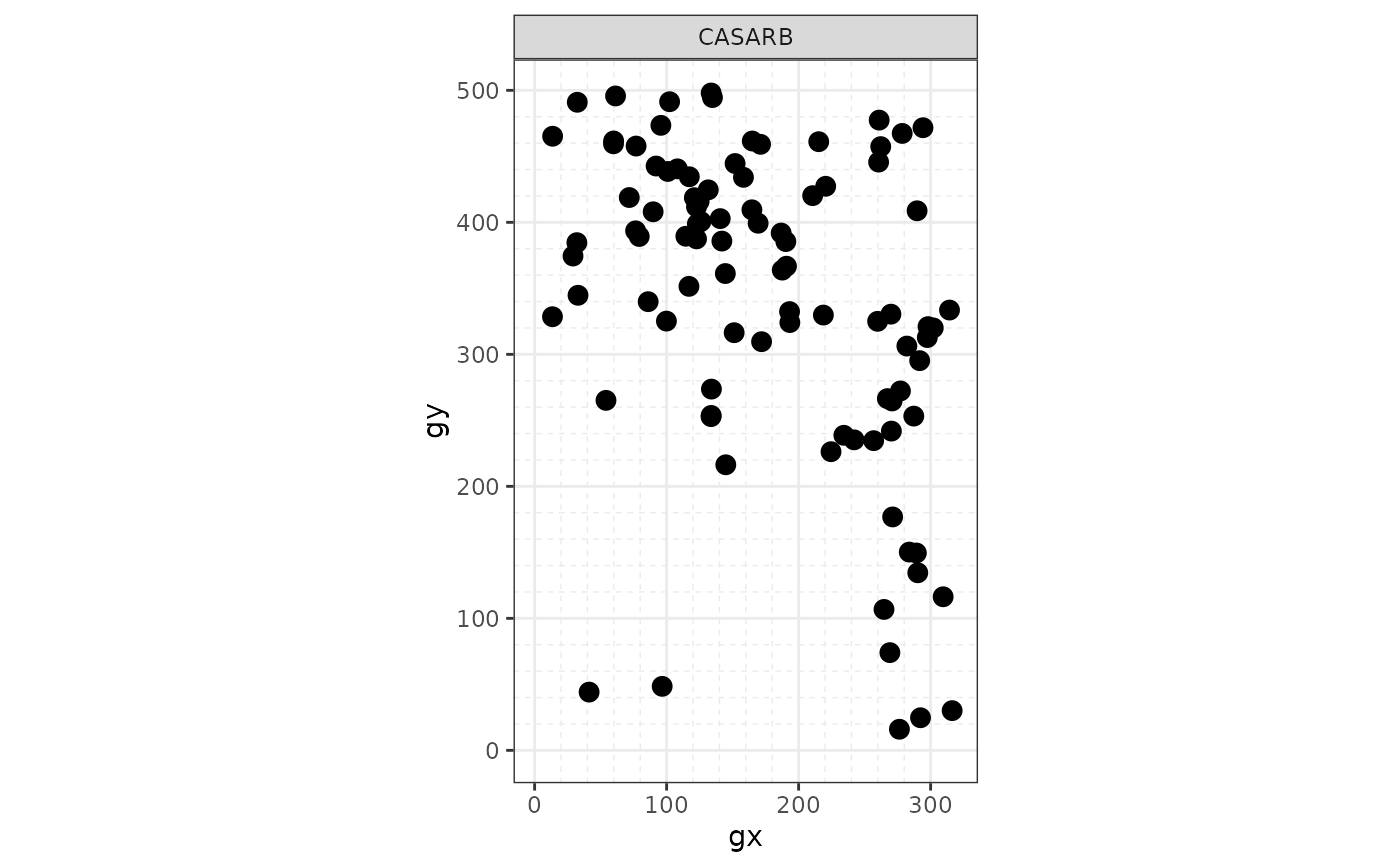#> #>$PREMON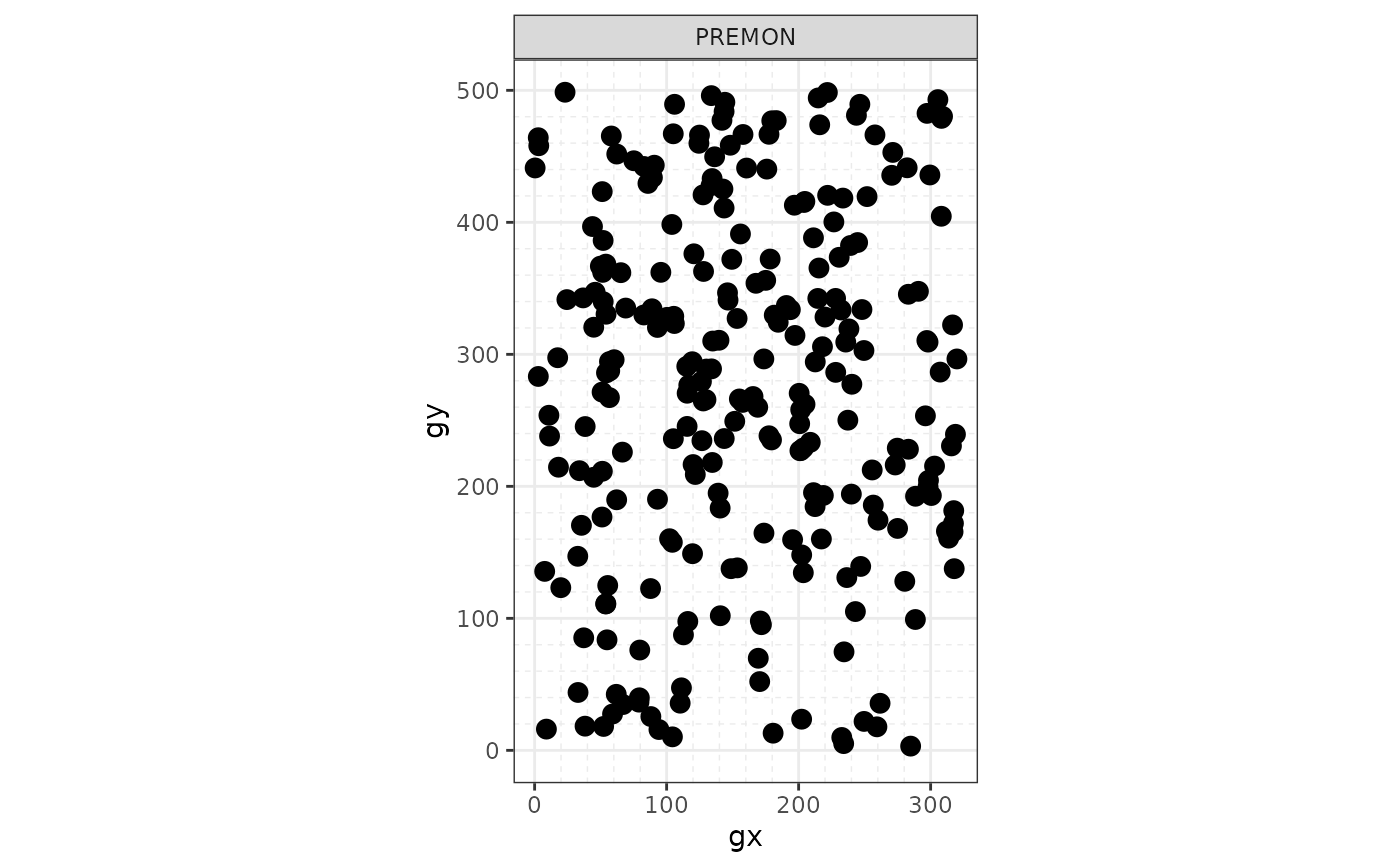#>
# To print all plots in a .pdf see ?pdf()
autoplot_by_species(sp(census))#> $CASARB#> #>$PREMON#>
#> $SLOBER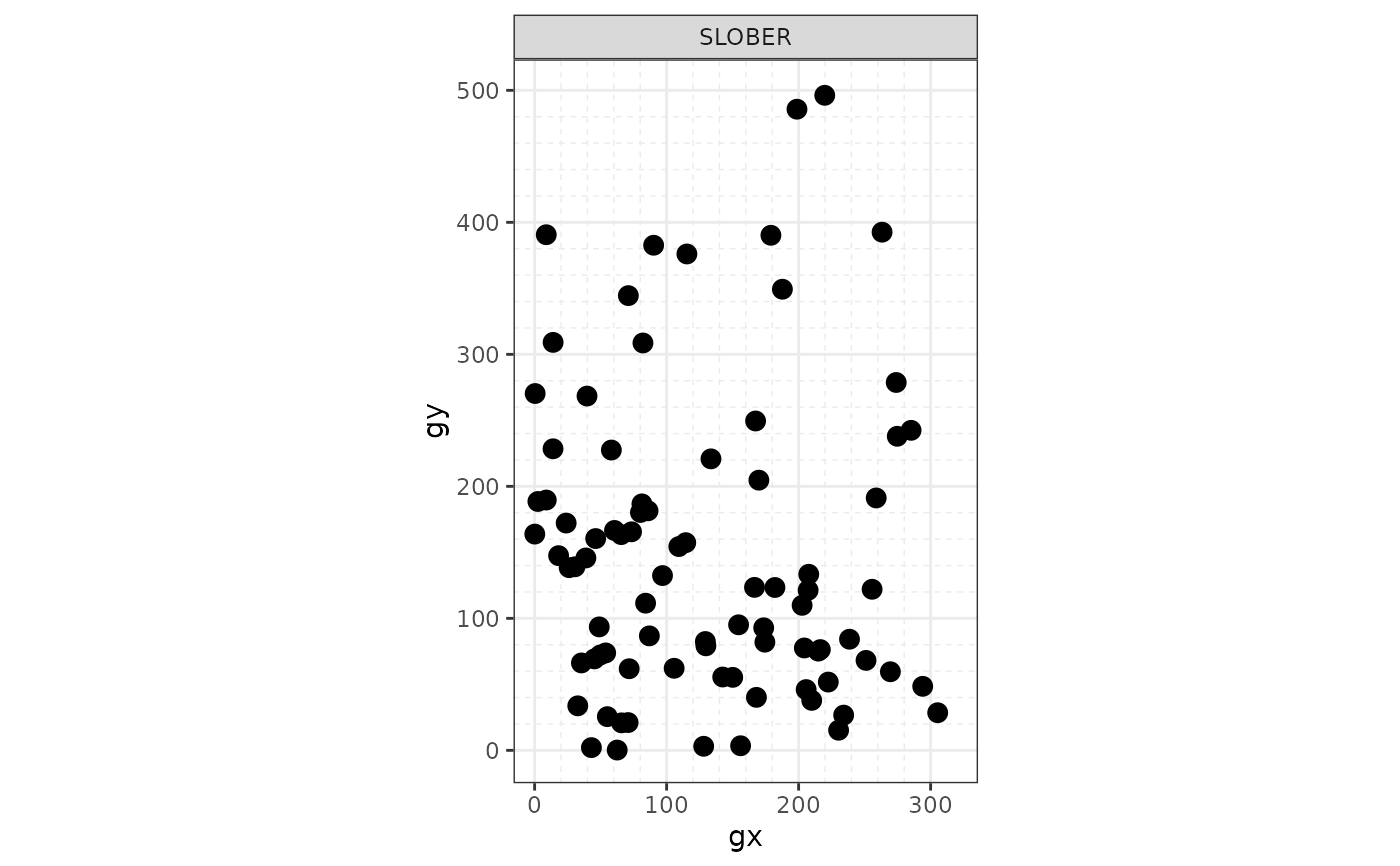#> # Species and elevation (optional) --------------------------------------- # Species and elevation elevation <- fgeo.x::elevation autoplot_by_species(sp_elev(census, elevation))#>$CASARB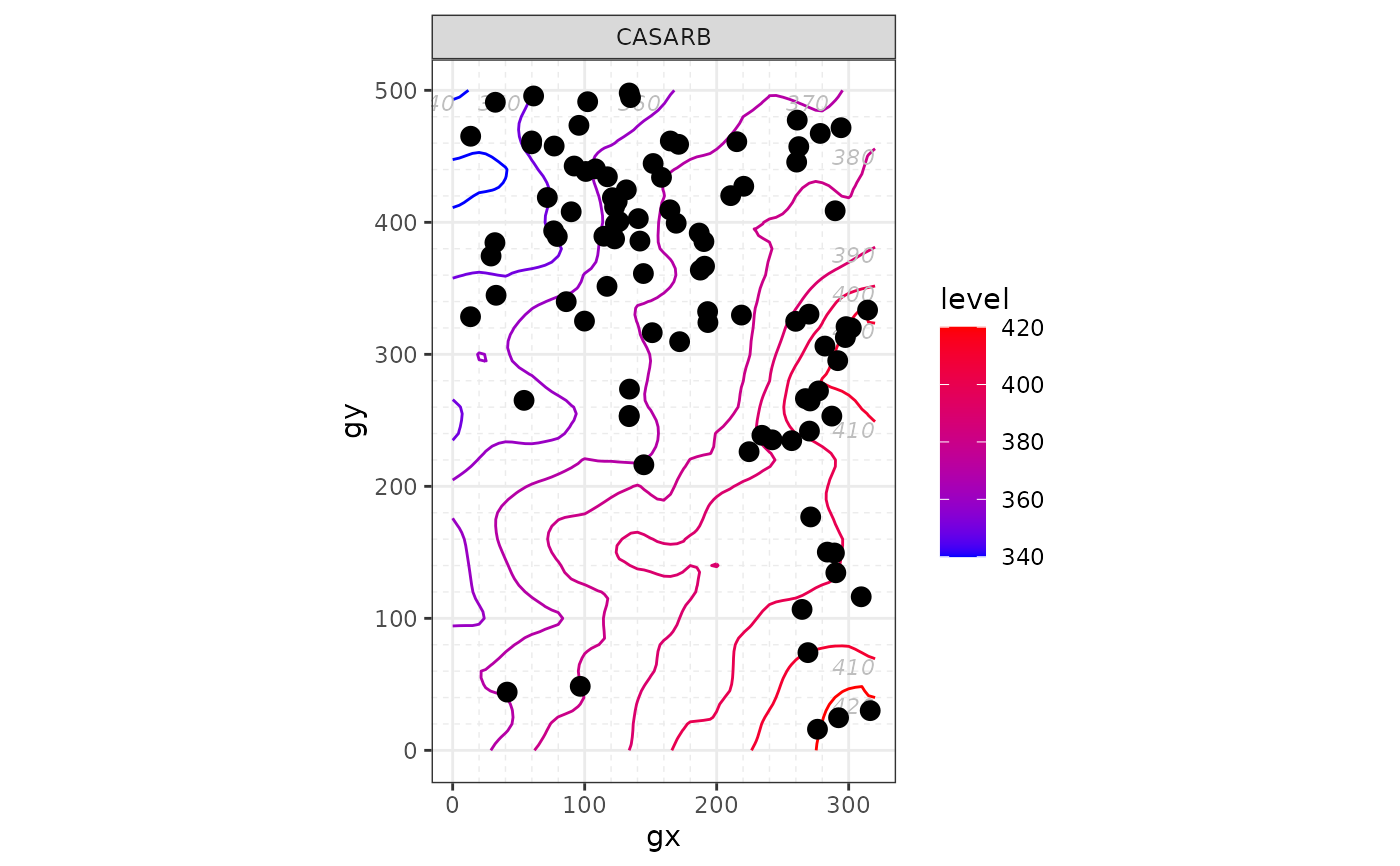#>
#> $PREMON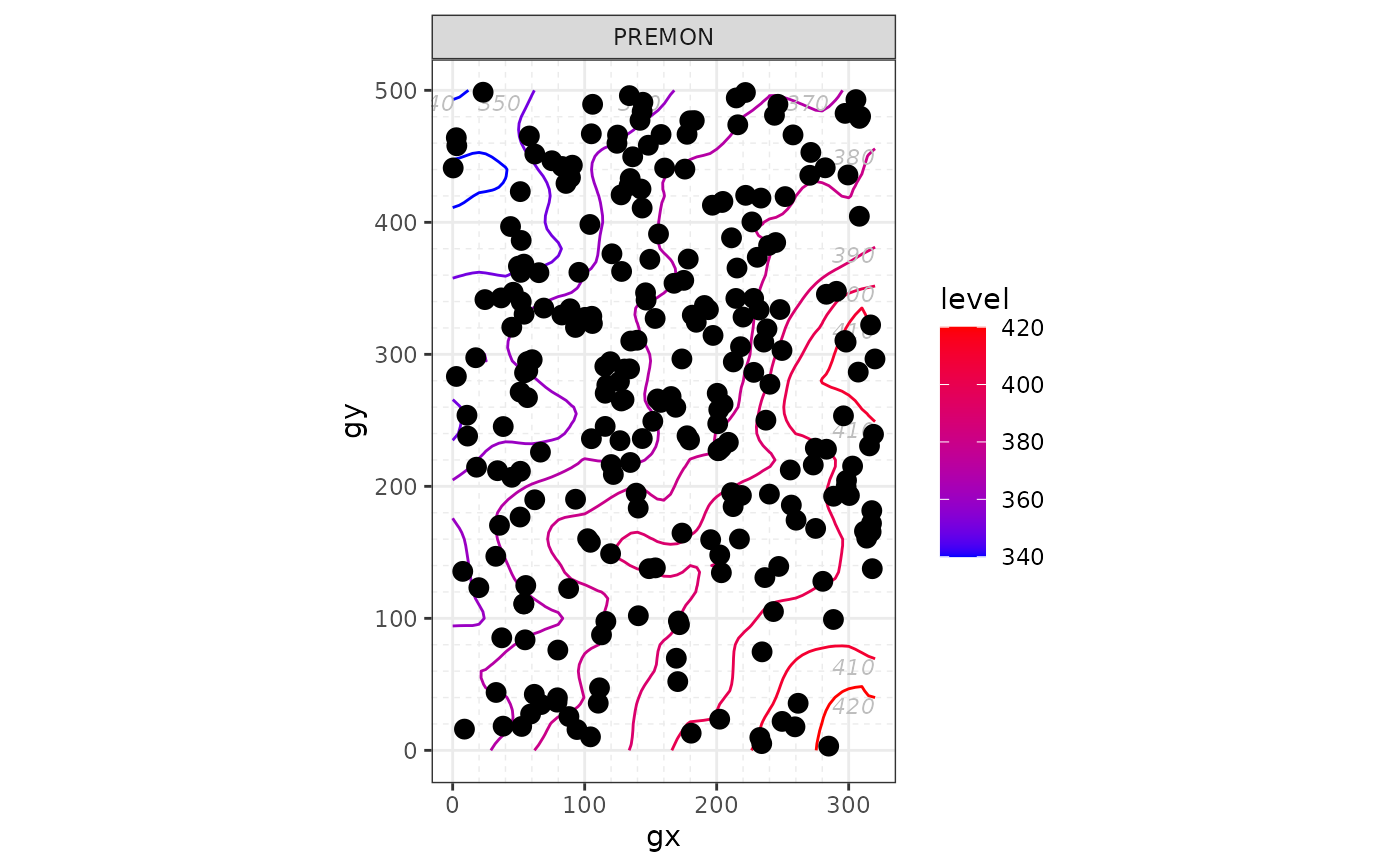#> #>$SLOBER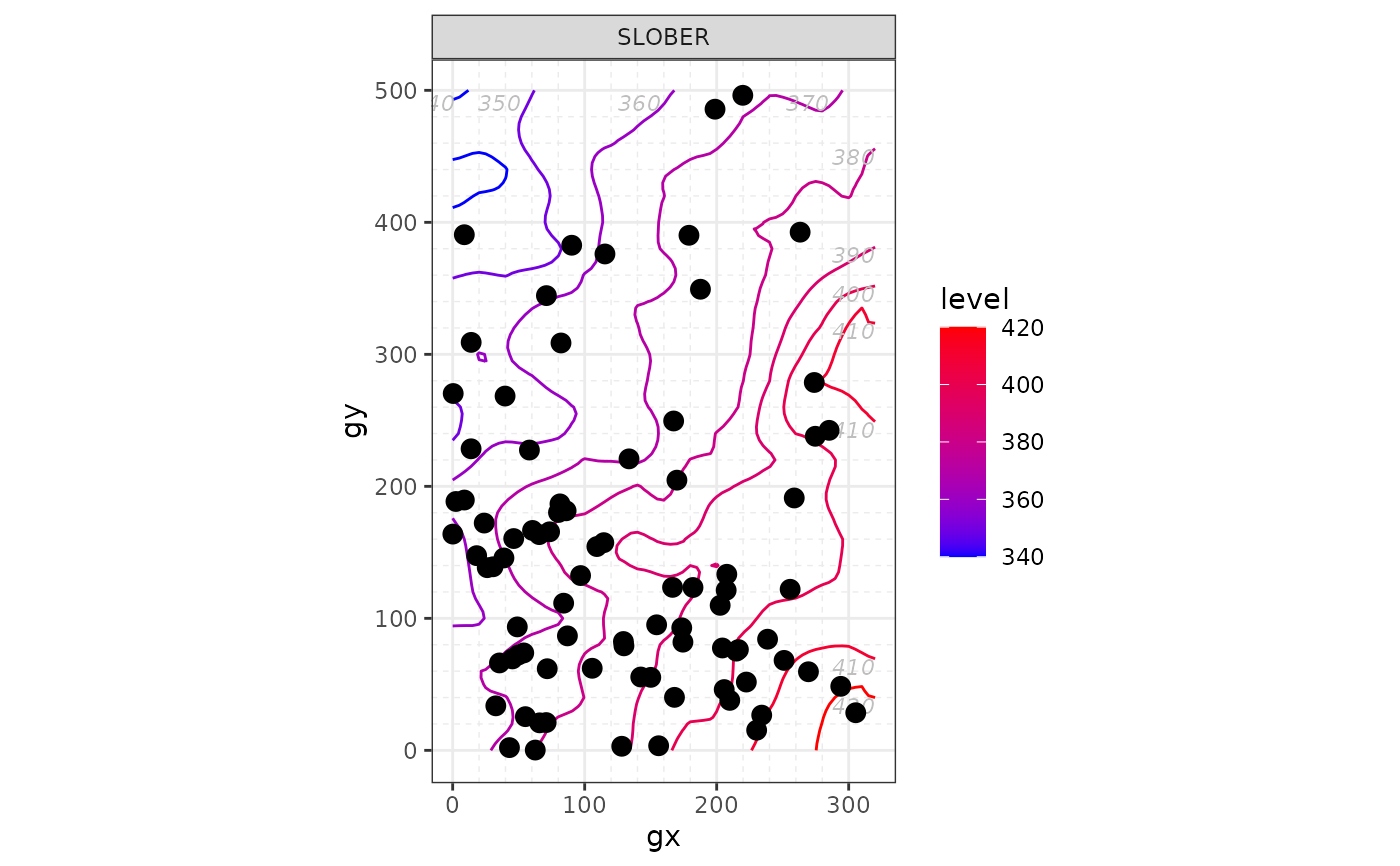#>